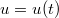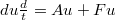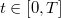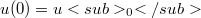ď»ż

### Introduzione

#### Abstract

En
We give a method to establish when the solution$u=u(t)$ of the semi-linear initial-value problem:${du \frac dt} = Au + Fu$$t \in [0,T]$;$u(0)=u_0$ belongs to a closed cone Q of a Banach space X.

DOI Code: §

Full Text: PDF